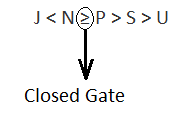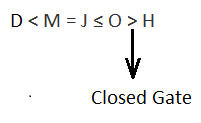Question of The Day16-06-2022

In the following question, relationship between different elements is shown in the statements. The statements are followed by two conclusions. Study the conclusions based on the given statements and select the appropriate answer.

Statement 1: D < M = J ≤ O > H;

Statement 2: J < N ≥ P > S > U;

Conclusion I: D < P

Conclusion II: H > U

Correct Answer : c ) Neither conclusion 1 nor conclusion 2 is true

Explanation :

Given Statements are,

Statement 1:  D < M = J ≤ O > H

Statement 2:  J < N ≥ P > S > U

Verifying conclusion I

Conclusion I: D < P

Using the given statements we will try to go to D from P through open gates, i.e. >, = and ≥. If we can reach D without seeing any closed gate, i.e., < and ≤, then our conclusion follows.

We can see in statement 2, that If we go from P to D, we need to go from P to J in statement 2 and from J to D in statement 1.

But we can see a closed gate while going from P to J in statement 1, so we cannot go to the next element from P.Thus, Conclusion I does not follow.

Verifying Conclusion II

Conclusion II: H > U

Using the given statements we will try to go to U from H through open gates, i.e. >, = and ≥. If we can reach U without seeing any closed gate, i.e., < and ≤ then our conclusion follows.

We can see in statement 1, that If we go from H to U, we need to go from H to J in statement 1 and from J to U in statement 2.

But we can see a closed gate while going from H to J in statement 1, so we cannot go to the next element from H.Thus, Conclusion II does not follow.

Hence, C is the correct answer.0